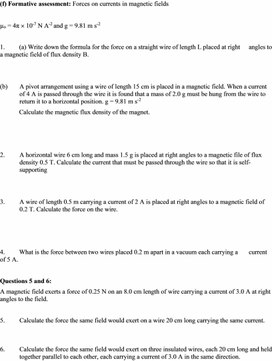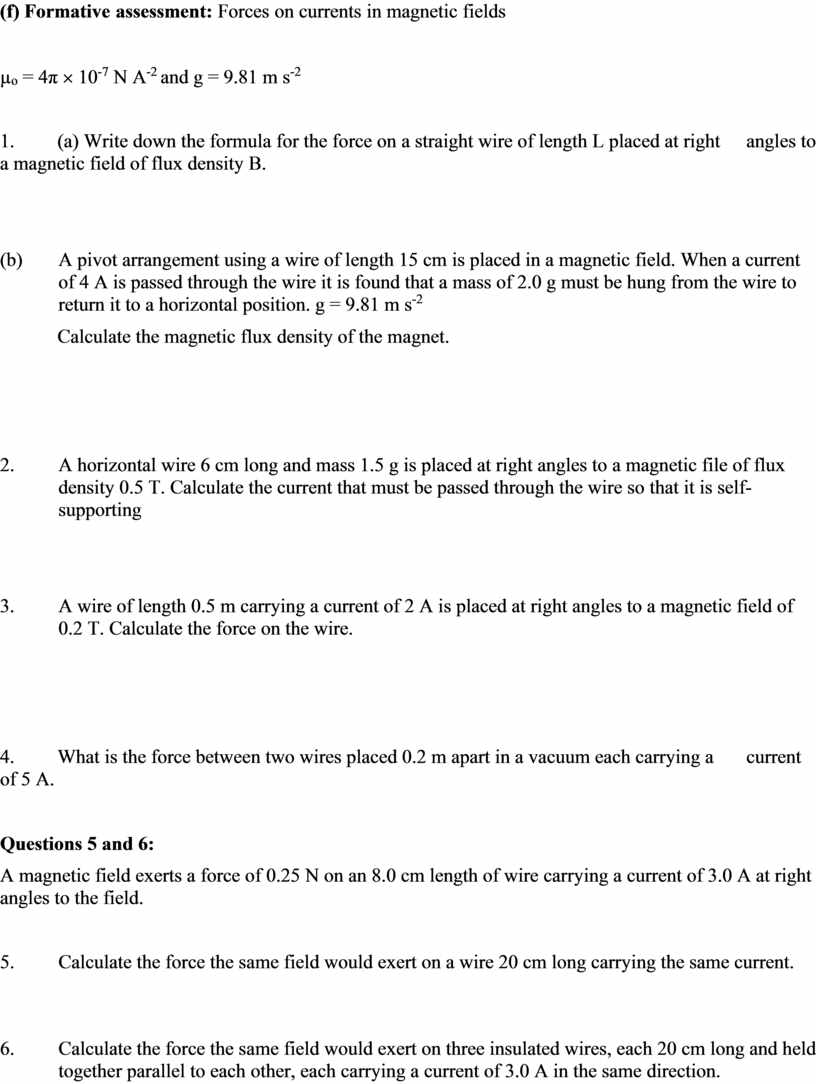PHY_10_5_V2_DM1_Ampere force. Left-hand rule
Оценка 5

# PHY_10_5_V2_DM1_Ampere force. Left-hand rule

Оценка 5
doc
07.05.2020PHY_10_5_V2_DM1_Ampere force. Left-hand rule.doc

(f) Formative assessment: Forces on currents in magnetic fields

µo = 4π ´ 10-7 N A-2 and g = 9.81 m s-2

1.         (a) Write down the formula for the force on a straight wire of length L placed at right        angles to a magnetic field of flux density B.

(b)       A pivot arrangement using a wire of length 15 cm is placed in a magnetic field. When a current of 4 A is passed through the wire it is found that a mass of 2.0 g must be hung from the wire to return it to a horizontal position. g = 9.81 m s-2

Calculate the magnetic flux density of the magnet.

2.         A horizontal wire 6 cm long and mass 1.5 g is placed at right angles to a magnetic file of flux density 0.5 T. Calculate the current that must be passed through the wire so that it is self-supporting

3.         A wire of length 0.5 m carrying a current of 2 A is placed at right angles to a magnetic field of 0.2 T. Calculate the force on the wire.

4.         What is the force between two wires placed 0.2 m apart in a vacuum each carrying a         current of 5 A.

Questions 5 and 6:

A magnetic field exerts a force of 0.25 N on an 8.0 cm length of wire carrying a current of 3.0 A at right angles to the field.

5.         Calculate the force the same field would exert on a wire 20 cm long carrying the same current.

6.         Calculate the force the same field would exert on three insulated wires, each 20 cm long and held together parallel to each other, each carrying a current of 3.0 A in the same direction.

## Formative assessment : Forces on currents in magnetic fields µ o = 4π ´ 10 -7Скачать файл